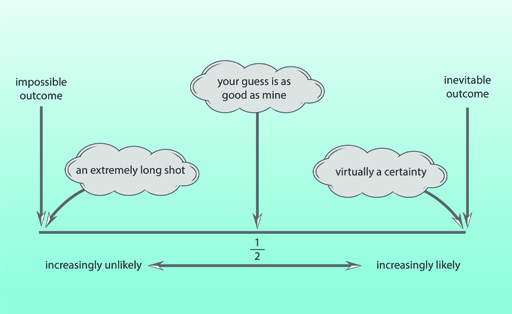Mathematics for science and technology

Start this free course now. Just create an account and sign in. Enrol and complete the course for a free statement of participation or digital badge if available.

Free course

# 2 Calculating probability

If a process is repeated in identical fashion a very large number of times, the probability of a given outcome is defined as the fraction of the results corresponding to that particular outcome.

Equation label: (1)

The nature of the fraction in Equation 1 shows that the probability of any given outcome cannot be smaller than 0 or larger than 1. A probability of 0 represents impossibility, while a probability of 1 represents inevitability. The closer the probability of a given outcome is to 1, the more likely that outcome is to occur. This is illustrated diagrammatically in Figure 1.Figure 1 The scale of probabilities

When a coin is tossed fairly, the likelihood of it landing on heads is equal to the likelihood of it landing on tails. If it is tossed repeatedly a great many times, it will in theory come up heads half the time: the probability of tossing heads is . The theoretical probability of tossing tails is, of course, also . The sum of these two probabilities ; i.e. it is certain that when the coin is tossed it will land either on heads or on tails. This is an example of a general rule:

The sum of the probabilities of all possible outcomes is equal to 1. A probability of 1 represents certainty.

Dice games involve rolling six-sided dice (singular ‘die’). Each face of a die is marked with a different score: one, two three, four, five or six. If the die is not loaded and the rolling is done fairly, then all outcomes are equally likely, so the probability of any one of the six possible outcomes (for example scoring a three) is . Again, the sum of the probabilities of all the possible outcomes is

So on one roll of the die the probability of scoring a three is and the probability of not scoring a three is . Another way of expressing this is to say that on a single roll of the die there is only one way of scoring a three, but there are 5 ways of not scoring a three. Clearly, it is more likely than not that a number other than three will be scored. This is just one illustration of another general rule:

The most likely outcome is the one that can occur in the greatest number of ways.

Provided nothing biases the result to make one outcome inherently more likely than others, the definition given by Equation 1 can be rewritten to encompass the number of ways in which a particular outcome may come about:

Equation label: (2)

Use these ideas in this activity.

## Activity 1 Calculating probability

1. What would be the probability of throwing an odd number on one roll of a die?

There are three possible ways of getting an odd number (1, 3 or 5) and six possible outcomes in total, so Equation 2 shows that the probability of throwing an odd number is , which can be simplified to the equivalent fraction . An alternative way of arriving at this conclusion is to say that as three of the possible outcomes are even and three are odd, the chances of one throw resulting in an odd number are the same as of it resulting in an even number. Hence the probability of an odd number is .

2.What is the probability of one card drawn at random from a shuffled pack of playing cards being: (a) a heart, (b) red, (c) an ace, (d) a picture card?

Note: if you are unfamiliar with playing cards, you need the following information. There are 52 cards in a pack, divided into four suits: hearts (red), diamonds (red), spades (black) and clubs (black). Each suit contains 13 cards, made up of one ace, nine ‘number’ cards (from 2 to 10 inclusive) and three picture cards (Jack, Queen, King).

• a.Of the 52 cards in the pack, 13 are hearts. So according to Equation 2, the probability of a card drawn at random being a heart is

{This result also follows from noting that there are 4 suits, each with the same number of cards, so one-quarter will be hearts.}

• b.Of the 52 cards in the pack, 26 are red (13 hearts and 13 diamonds). So the probability of a card drawn at random being red is

{Alternatively 2 of the 4 suits are red, so the probability is .}

• c.Of the 52 cards in the pack, 4 are aces (one for each suit). So the probability of a card drawn at random being an ace is

• d.Of the 52 cards in the pack, 12 are picture cards (3 for each suit). So the probability of a card drawn at random being a picture card is

But what does a probability mean in real life?

MST_1